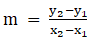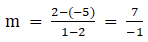• +91 9971497814
• info@interviewmaterial.com

# RD Chapter 23- The Straight Lines Ex-23.3 Interview Questions Answers

### Related Subjects

Question 1 : Find the equation of a line making an angle of 150° with the x–axis and cutting off an intercept 2 from y–axis.

Given: A line whichmakes an angle of 150o with the x–axis and cutting off anintercept at 2

By using the formula,

The equation of a lineis y = mx + c

We know thatangle, θ = 150o

The slope of the line,m = tan θ

Where, m = tan 150o

= -1/ √3

Coordinate ofy–intercept is (0, 2)

The required equationof the line is y = mx + c

Now substitute thevalues, we get

y = -x/√3 + 2

3y – 2√3 + x = 0

x + √3y = 2√3

The equation of lineis x + √3y = 2√3

Question 2 :
Find the equation of a straight line:
(i) with slope 2 and y – intercept 3;
(ii) with slope – 1/ 3 and y – intercept – 4.
(iii) with slope – 2 and intersecting the x–axis at a distance of 3 units to the left of origin.

(i) With slope 2 and y –intercept 3

The slope is 2 and thecoordinates are (0, 3)

Now, the requiredequation of line is

y = mx + c

Substitute the values,we get

y = 2x + 3

(ii) With slope – 1/ 3 andy – intercept – 4

The slope is – 1/3 andthe coordinates are (0, – 4)

Now, the requiredequation of line is

y = mx + c

Substitute the values,we get

y = -1/3x – 4

3y + x = – 12

(iii) With slope – 2 andintersecting the x–axis at a distance of 3 units to the left of origin

The slope is – 2 andthe coordinates are (– 3, 0)

Now, the requiredequation of line is y – y1 = m (x – x1)

Substitute the values,we get

y – 0 = – 2(x + 3)

y = – 2x – 6

2x + y + 6 = 0

Question 3 : Find the equations of the bisectors of the angles between the coordinate axes.

There are two bisectorsof the coordinate axes.

Their inclinationswith the positive x-axis are 45o and 135o

The slope of thebisector is m = tan 45o or m = tan 135o

i.e., m = 1 or m = -1,c = 0

By using the formula,y = mx + c

Now, substitute thevalues of m and c, we get

y = x + 0

x – y = 0 or y = -x +0

x + y = 0

The equation of thebisector is x ± y = 0

Question 4 : Find the equation of a line which makes an angle of tan – 1 (3) with the x–axis and cuts off an intercept of 4 units on the negative direction of y–axis.

Given:

The equation whichmakes an angle of tan – 1(3) with the x–axis and cuts off anintercept of 4 units on the negative direction of y–axis

By using the formula,

The equation of theline is y = mx + c

Here,angle θ = tan – 1(3)

So, tan θ = 3

The slope of the lineis, m = 3

And, Intercept in thenegative direction of y–axis is (0, -4)

The required equationof the line is y = mx + c

Now, substitute thevalues, we get

y = 3x – 4

The equation of theline is y = 3x – 4.

Question 5 : Find the equation of a line that has y – intercept – 4 and is parallel to the line joining (2, –5) and (1, 2).

Given:

A line segment joining(2, – 5) and (1, 2) if it cuts off an intercept – 4 from y–axis

By using the formula,

The equation of lineis y = mx + C

It is given that, c =– 4

Slope of line joining(x1 – x2) and (y1 – y2),So, Slope of linejoining (2, – 5) and (1, 2),m = – 7

The equation of lineis y = mx + c

Now, substitute thevalues, we get

y = –7x – 4

y + 7x + 4 = 0

The equation of lineis y + 7x + 4 = 0.

Todays Deals### RD Chapter 23- The Straight Lines Ex-23.3 Contributorskrishan

Name:
Email:

# Latest News# 9000 interview questions in different categories